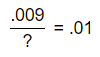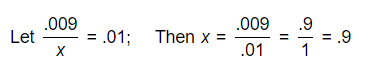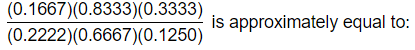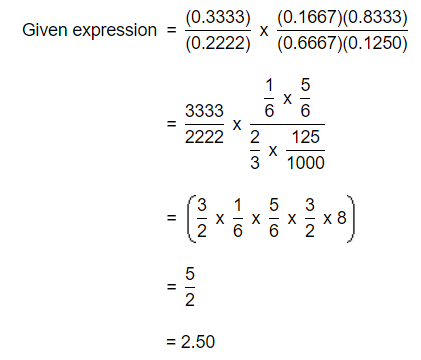Decimal Fractions MCQ Test

# Decimal Fractions MCQ Test

Test Description

## 5 Questions MCQ Test UPSC Prelims Paper 2 CSAT - Quant, Verbal & Decision Making | Decimal Fractions MCQ Test

Decimal Fractions MCQ Test for Railways 2023 is part of UPSC Prelims Paper 2 CSAT - Quant, Verbal & Decision Making preparation. The Decimal Fractions MCQ Test questions and answers have been prepared according to the Railways exam syllabus.The Decimal Fractions MCQ Test MCQs are made for Railways 2023 Exam. Find important definitions, questions, notes, meanings, examples, exercises, MCQs and online tests for Decimal Fractions MCQ Test below.
Solutions of Decimal Fractions MCQ Test questions in English are available as part of our UPSC Prelims Paper 2 CSAT - Quant, Verbal & Decision Making for Railways & Decimal Fractions MCQ Test solutions in Hindi for UPSC Prelims Paper 2 CSAT - Quant, Verbal & Decision Making course. Download more important topics, notes, lectures and mock test series for Railways Exam by signing up for free. Attempt Decimal Fractions MCQ Test | 5 questions in 10 minutes | Mock test for Railways preparation | Free important questions MCQ to study UPSC Prelims Paper 2 CSAT - Quant, Verbal & Decision Making for Railways Exam | Download free PDF with solutions
 1 Crore+ students have signed up on EduRev. Have you?
Decimal Fractions MCQ Test - Question 1

### When 0.232323..... is converted into a fraction, then the result is:

Detailed Solution for Decimal Fractions MCQ Test - Question 1

Explanation:Decimal Fractions MCQ Test - Question 2

###Detailed Solution for Decimal Fractions MCQ Test - Question 2

Explanation:Decimal Fractions MCQ Test - Question 3

### The expression (11.98 * 11.98 + 11.98x + 0.02 * 0.02) will be a perfect square for x equal to:

Detailed Solution for Decimal Fractions MCQ Test - Question 3

Explanation:

Given expression = (11.98)2 + (0.02)2 + 11.98 x x.

For the given expression to be a perfect square, we must have

11.98 x x = 2 x 11.98 x 0.02 or x   = 0.04

Decimal Fractions MCQ Test - Question 4Detailed Solution for Decimal Fractions MCQ Test - Question 4

Explanation:Decimal Fractions MCQ Test - Question 5

3889 + 12.952 - ? = 3854.002

Detailed Solution for Decimal Fractions MCQ Test - Question 5

Explanation:

Let 3889 + 12.952 - x = 3854.002.

Then x = (3889 + 12.952) - 3854.002

= 3901.952 - 3854.002

= 47.95.

## UPSC Prelims Paper 2 CSAT - Quant, Verbal & Decision Making

67 videos|50 docs|151 tests
 Use Code STAYHOME200 and get INR 200 additional OFF Use Coupon Code
Information about Decimal Fractions MCQ Test Page
In this test you can find the Exam questions for Decimal Fractions MCQ Test solved & explained in the simplest way possible. Besides giving Questions and answers for Decimal Fractions MCQ Test, EduRev gives you an ample number of Online tests for practice

## UPSC Prelims Paper 2 CSAT - Quant, Verbal & Decision Making

67 videos|50 docs|151 tests